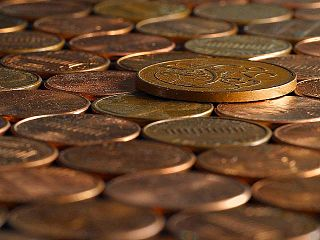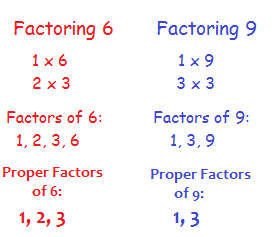# What is an Abundant Number?

Instructor: Danielle Wilson

Danielle is a certified elementary, middle school math, and special education teacher. She has a master's degree in elementary education and special education.

There are so many things we can do with numbers. We can add them, multiply them, find their square roots and much more. In this lesson, you will learn what an abundant number is and how to figure out if a number is abundant.

## Large Quantity

Could you imagine having an abundance of money? What would you buy? Would you go on a long beach vacation? Or would you buy all of the video games you've always wanted? When you have an abundance of something, it means you have a large amount. So then, what exactly is an abundant number?An abundant number is a number where the sum of all its proper factors is larger than the original number. Abundant numbers are cool - you can think of them as being made up of more than what they're worth! Abundant numbers are a lot like U.S. pennies. It costs about 1.7 cents to make a single penny, but all pennies are only worth one cent! Hard to believe, right?

## Finding Abundant Numbers

In order to determine if a number is abundant, you must first find all of the number's proper factors. While factors are numbers that you multiply together to get another number, proper factors are all the factors of the number except the number itself.Once you have found all of the proper factors of a number, you add them together. If the sum of the proper factors is greater than your actual number, then the number is considered to be an abundant number.

The first abundant number is 12. The proper factors of 12 are 1, 2, 3, 4, and 6. Now that we have factored, let's add the factors together. If you add 1 + 2 + 3 + 4 + 6 you will get 16. The number 16 is greater than the number 12, so 12 is an abundant number. The difference between the sum of the factors and the original number is known as the abundance. The abundance of 12 is 4, because 16 - 12 = 4.

## Now You Try

Is 24 an abundant number?

#### Step 1: List all of the proper factors of 24 (remember to not include 24):

1, 2, 3, 4, 6, 8, 12

#### Step 2: Add the factors together.

1 + 2 + 3 + 4 + 6 + 8 + 12 = 36

Since 36 is greater than 24, 24 is also an abundant number. 36 - 24 = 8 so the abundance of 24 is 8.

Okay, now I want you to grab a pencil and paper and figure out if 48 is abundant, and if it is, find the abundance.

Remember to first list the proper factors of the number! Be sure not to scroll down and peek at the answer.

To unlock this lesson you must be a Study.com Member.

### Register to view this lesson

Are you a student or a teacher?

#### See for yourself why 30 million people use Study.com

##### Become a Study.com member and start learning now.
Back
What teachers are saying about Study.com

### Earning College Credit

Did you know… We have over 200 college courses that prepare you to earn credit by exam that is accepted by over 1,500 colleges and universities. You can test out of the first two years of college and save thousands off your degree. Anyone can earn credit-by-exam regardless of age or education level.